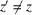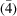International
Tables for
Crystallography
Volume B
Reciprocal space
Edited by U. Shmueli

International Tables for Crystallography (2010). Vol. B, ch. 2.5, pp. 308-309   | 1 | 2 |

## Section 2.5.3.2.1. Symmetry elements of a specimen and diffraction groups

M. Tanakaf

#### 2.5.3.2.1. Symmetry elements of a specimen and diffraction groups

| top | pdf |

Since CBED uses the Laue geometry, Buxton et al. (1976) assumed a perfectly crystalline specimen in the form of a parallel-sided slab which is infinite in two dimensions. The symmetry elements of the specimen (as distinct from those of an infinite crystal) form `diffraction groups', which are isomorphic to the point groups of the diperiodic plane figures and Shubnikov groups of coloured plane figures. The diffraction groups of a specimen are determined from the symmetries of CBED patterns taken at various orientations of the specimen. The crystal point-group of the specimen is identified by referring to Fig. 2.5.3.4, which gives the relation between diffraction groups and crystal point groups.

A specimen that is parallel-sided and is infinitely extended in the x and y directions has ten symmetry elements. The symmetry elements consist of six two-dimensional symmetry elements and four three-dimensional ones. The operation of the former elements transforms an arbitrary coordinate (x, y, z) into (x′, y′, z), with z remaining the same. The operation of the latter transforms a coordinate (x, y, z) into (x′, y′, z′), where. A vertical mirror plane m and one-, two-, three-, four- and sixfold rotation axes that are parallel to the surface normal z are the two-dimensional symmetry elements. A horizontal mirror plane m′, an inversion centre i, a horizontal twofold rotation axis 2′ and a fourfold rotary inversionare the three-dimensional symmetry elements, and are shown in Fig. 2.5.3.1. The fourfold rotary inversion was not recognized as a symmetry element until the point groups of the diperiodic plane figures were considered (Buxton et al., 1976). Table 2.5.3.1lists these symmetry elements, where the symbols in parentheses express symmetries of CBED patterns expected from three-dimensional symmetry elements.

 Table 2.5.3.1| top | pdf | Two- and three-dimensional symmetry elements of an infinitely extended parallel-sided specimen
 Symbols in parentheses show CBED symmetries appearing in dark-field patterns.
Two-dimensional symmetry elementsThree-dimensional symmetry elements
1 m′ (1R)
2 i (2R)
3 2′ (m2, mR)
4(4R)
5
6
mFigure 2.5.3.1 | top | pdf |Four symmetry elements m′, i, 2′ andof an infinitely extended parallel-sided specimen.

The diffraction groups are constructed by combining these symmetry elements (Table 2.5.3.2). Two-dimensional symmetry elements and their combinations are given in the top row of the table. The third symmetry m in parentheses is introduced automatically when the first two symmetry elements are combined. Three-dimensional symmetry elements are given in the first column. The equations given below the table indicate that no additional three-dimensional symmetry elements can appear by combination of two symmetry elements in the first column. As a result, 31 diffraction groups are produced by combining the elements in the first column with those in the top row. Diffraction groups in square brackets have already appeared ealier in the table. In the fourth row, three columns have two diffraction groups, which are produced when symmetry elements are combined at different orientations. In the last row, five columns are empty because a fourfold rotary inversion cannot coexist with threefold and sixfold rotation axes. In the last column, the number of independent diffraction groups in each row is given, the sum of the numbers being 31.

 Table 2.5.3.2| top | pdf | Symmetry elements of an infinitely extended parallel-sided specimen and diffraction groups
12346m2m(m)3m4m(m)6m(m)
1 1 2 3 4 6 m 2m(m) 3m 4m(m) 6m(m) 10
(m′) 1R 1R 21R 31R 41R 61R m1R 2m(m)1R 3m1R 4m(m)1R 6m(m)1R 10
(i) 2R 2R [21R] 6R [41R] [61R] 2Rm(mR) [2m(m)1R] 6Rm(mR) [4m(m)1R] [6m(m)1R] 4
[2Rm(mR)] [2m(m)1R] [3m1R]
(2′) mR mR 2mR(mR) 3mR 4mR(mR) 6mR(mR) [m1R] [4R(m)mR] [6Rm(mR)] [4m(m)1R] [6Rm(mR)] 54R   4R   [41R]   4Rm(mR) [4Rm(mR)]   [4m(m)1R]   2

1R × 2R = 2, 2R × 2R = 1, mR × 2R = m, 4R × 2R = 4, 1R × mR = m × mR, 1R × 4R = 4 × 1R, mR × 4R = m × 4R.

### References

Buxton, B., Eades, J. A., Steeds, J. W. & Rackham, G. M. (1976). The symmetry of electron diffraction zone axis patterns. Philos. Trans. R. Soc. London Ser. A, 181, 171–193.